Next: 4. Ballistic Thermal Properties of Silicon-Based Nanostructures Up: 3.3 Thermoelectrics Engineering in ZGNRs Previous: 3.3.4 The Effect of Roughness on Phonon Transport   Contents

## 3.3.5 Thermoelectric Figure of Merit

Using the first design step, i.e. the effect of ELDs, it was demonstrated that the transmission of electrons around the Fermi level can be increased (from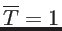to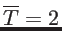and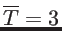in the presence of one and two ELDs, respectively). An asymmetry is thus created between holes and electrons. This increases both the conductance and the Seebeck coefficient of the channel as shown in Fig. 3.27. Figure 3.27-a shows the conductance of the 2ELD-ZGNR(8,4,8) (blue), of the ELD-ZGNR (10,10) (red), and of the pristine nanoribbon (green) at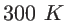. As expected, the conductance of the channel with two ELDs is the largest, followed by the channel with one ELD. The conductances are larger than that of the pristine channel by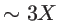and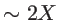, respectively. Figure 3.27-b shows the changes of the Seebeck coefficient after the introduction of the ELDs in the nanoribbon. Due to its metallic behavior and the flat transmission near the Fermi level, the pristine channel exhibits zero Seebeck coefficient. Due to the asymmetry built up after the introduction of the ELDs, however, the Seebeck coefficient increases for both channels. The channel with two line defects has the largest asymmetry, and therefore the largest Seebeck coefficient (in absolute values). Finally, the power factor in Fig. 3.27-c is indeed largely improved in the ELD structures, and especially the 2ELD-ZGNR channel.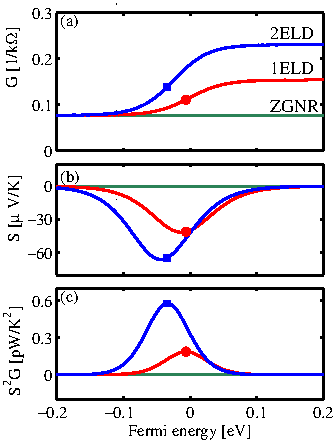In Figure 3.28 the same thermoelectric coefficients for the same structures as in Fig. 3.27 are shown, but now edge roughness and positive impurities are included in the calculation. The length of the channels in this case is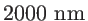. A similar qualitative behavior is observed as in Fig. 3.27 for both channels. Quantitatively, however, the conductance in Fig. 3.28-a is now significantly reduced by a factor of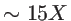(the dots correspond to the position of the peak of the power factor of the devices without roughness and impurities in Fig. 3.27). The Seebeck coefficient in Fig. 3.28-b, on the other hand increases. Finally, the peak of the power factor in Fig. 3.28-c reduces only slightly compared to the peak of the power factor of the devices without edge roughness in Fig. 3.27-c (dots).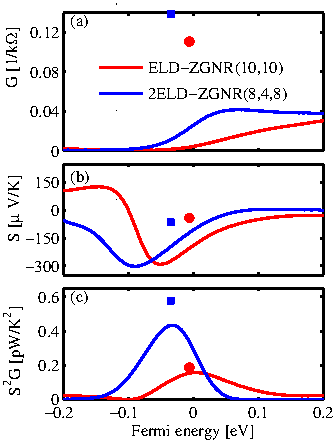For the devices that include rough edges, however, as demonstrated in Fig. 3.26, the phonon thermal conductivity is drastically reduced compared to the electronic thermal conductivity. A large improvement in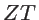is therefore expected. Figure 3.29 showsversus energy at room temperature for the ELD-ZGNR(10,10), the ELD-ZGNR(10,10) with impurities and roughness (red), and the 2ELD-ZGNR(8,4,8) (blue) with impurities and roughness. As indicated, large values ofcan be achieved, especially in the case of the device with two ELDs. The phonon lattice conductivity value used in this calculation was extracted using the MFP method. As explained in Sec. 3.3.4, that value could belower than the value extracted from direct integration of the simulated phonon transmission. In the inset of Fig. 3.29 theversus energy using the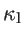values extracted from the transmission are shown. Indeed the values might be reduced by a factor of, but still peakvalues above 2 can be achieved at room temperature, which is comparable and even better than the best thermoelectric materials to date . It is worth mentioning that as shown by Ref.  rough ZGNRs can have highvalues even without the presence of ELDs. For this the asymmetry in the sharp edges of the higher subbands is utilized at energies above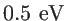. Those energies however, are too high and can not easily be reached. Finally it is necessary to mention here that the formalism has considered scattering only by edge roughness and impurity scattering, whereas phonon scattering and dephasing mechanisms are not included. However, as it is shown for 1D nanowires , the effects of impurity scattering and edge roughness are the most important scattering effects in channels of cross sections below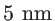, and one can expect this to hold also for GNRs as well.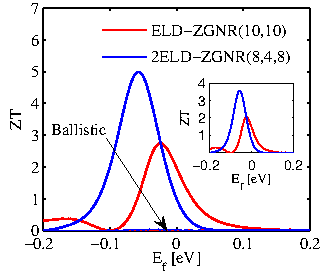Next: 4. Ballistic Thermal Properties of Silicon-Based Nanostructures Up: 3.3 Thermoelectrics Engineering in ZGNRs Previous: 3.3.4 The Effect of Roughness on Phonon Transport   Contents
H. Karamitaheri: Thermal and Thermoelectric Properties of Nanostructures